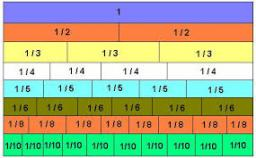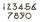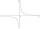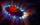# Unknown number

I think the number - its sixth is 3 smaller than its third.

Correct result:

x =  18

#### Solution:

x/6 = x/3 - 3

x = 18

Our simple equation calculator calculates it.We would be pleased if you find an error in the word problem, spelling mistakes, or inaccuracies and send it to us. Thank you!Tips to related online calculators
Need help calculate sum, simplify or multiply fractions? Try our fraction calculator.
Do you have a linear equation or system of equations and looking for its solution? Or do you have quadratic equation?

## Next similar math problems:

• Unknown numberI think the number. I'll reduce it to its one-third. The result is then increased by one-third, and I get the number 12.
• NumberWhat number I think? The third is 6 and half is 2 more than the its quarter.
• Unknown number 6Determine the unknown number, which is by 1.5 greater than its fourth.
• NumberI think the number. If I add to its third seven I get same as when to its quarter add 8. Which is the number?
• Unknown number 24I think the number: a - is the same as the square area that has the 12th circumfence. What is this number? b - its half is 7 times bigger than its quarter. Is this the number?
• Unknown mixed numberFind the number that is smaller than 5 5/12 by as much as 2 2/13 is smaller than 6 1/6
• Unknown number 5I think unknown number. If we enlarge it five times then subtract 3 and result decreases by 75% we get the number by one greater than the number I think. What number am I thinking?
• Unknown numberDetermine the unknown number that is equal to a quarter of the fifth of number, which is by 152 more than unknown number.
• 15 numberWhat number is smaller (greater) by 15 than its half?
• Unknown number 5Daniel think an integer. When he change this number at a ratio of 2:5 he got number 2.8. Determine what number think Daniel.
• Sum of three numbersThe sum of three numbers from which second number is 20% smaller than the first number and the third number is 25% smaller than the second number is 96. Determine this numbers.
• Cleaning windowsCleaning company has to wash all the windows of the school. The first day washes one-sixth of the windows of the school, the next day three more windows than the first day and the remaining 18 windows washes on the third day. Calculate how many windows ha
• Ratio of two unknown numbersTwo numbers are given. Their sum is 30. We calculate one-sixth of a larger number and add to both numbers. So we get new numbers whose ratio is 5:7. Which two numbers were given?
• Unknown number 4I think number. When I divided its square root by its 1/9, I get a number 1. What number am I thinking?
• Algebra problemThis is algebra. Let n represent an unknown number. 1. Eight more than the number n 2. Three times the number n 3. The product of the number n and eight 4. Three less than the number n 5. Three decreased by the number n
• FamilySon is 3/5 smaller than the mother. Mother is 99 cm higher than the son. How tall is the mother?
• Expressions with variableThis is algebra. Let n represent an unknown number and write the following expressions: 1. 4 times the sum of 7 and the number x 2. 4 times 7 plus the number x 3. 7 less than the product of 4 and the number x 4. 7 times the quantity 4 more than the number